### Home > PC > Chapter 1 > Lesson 1.3.2 > Problem1-116

1-116.
1.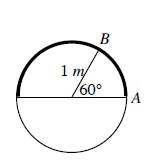The circle to the right has a radius of one meter. Homework Help ✎

1. How many degrees are in a half circle?

2. What is the length of the arc that covers half of the circle?

3. Using exact values, what is the length of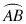? You will have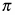as part of your answer.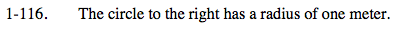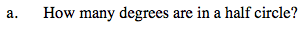It is half of 360° or 180°.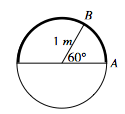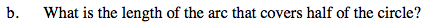Since the circumference of the circle is 2π(radius), the answer is 2π(1). So what is the arc length of half of the circle?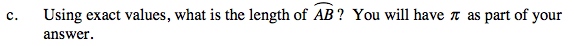What part of the circumference will 60° have?

$\frac{1}{6}\text{ of\ the\ circumference\ or\ }\frac{2\pi}{6}$